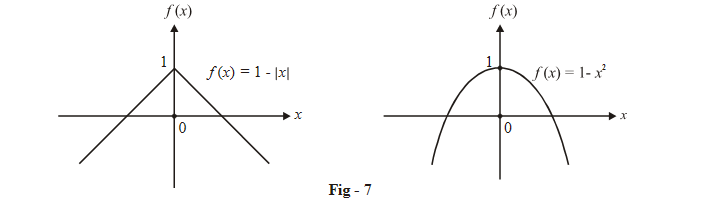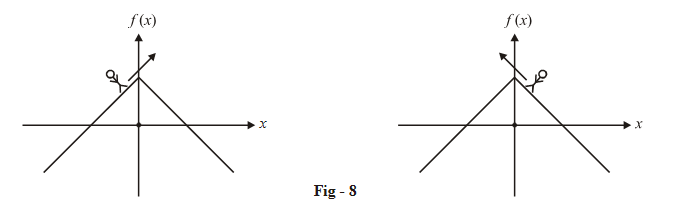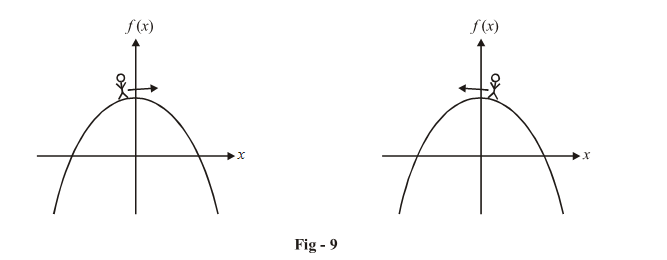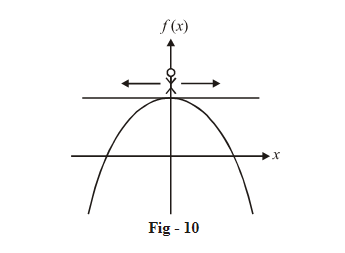# Introduction To Differentiability

Go back to  'LCD'

Having seen the concept and the physical (graphical) significance of continuity, we now turn our attention to the concept of differentiability.

Consider the two graphs given in the figure below:What is the striking difference between the two graphs at the origin (apart from one being linear and the other, non-linear)? The first has a sharp, sudden turn at the y-axis while the second passes ‘smoothly’ through the y-axis.

Lets make this idea more concrete. Imagine a person called Theta walking on the graph of  f (x) = 1– |x|, towards the y-axis, once from the left and once from the right:While walking from the left, Theta will be moving in a north-east direction as he approaches the y-axis; while walking from the right, he will be walking in a north-west direction.

Now consider Theta walking on $$f\left( x \right){\rm{ }} = {\rm{ }}1{\rm{ }}-{x^2}$$ , once from the left and once from the right, towards  x = 0.As Theta approaches the y-axis, we see that he moves ‘almost’ horizontally near the y-axis, in both the cases. The line of travel becomes almost the same from either side near x = 0. At x = 0, the line of travel becomes precisely horizontal (for an instant), whether Theta is walking from the left or the right. (This unique line of travel is obviously the tangent drawn at x = 0)In mathematical language, since the lines of travel from both sides tend to become the same as the y-axis is approached or more precisely, the tangent drawn to the immediate left of x = 0 and the one to the immediate right, become precisely the same at x = 0 (a unique tangent), we say that the function $$f\left( x \right){\rm{ }} = {\rm{ }}1{\rm{ }}-{x^2}$$ is differentiable at x = 0. This means that the graph is “smoothly varying” around x = 0 or there is no sharp turn at x = 0.

In the case of f (x) = 1– |x|, the lines of travel from the left hand and the right hand sides are different. The line of travel (or tangent) to the immediate left of x = 0 is inclined at 45º to the x-axis while the one to the immediate right is inclined at 135º. Precisely at x = 0, there is no unique tangent that can be drawn to f (x). We therefore say that f (x) = 1 – |x| is non-differentiable at x = 0. This means that the graph has a sharp point (or turn) at x = 0, as is evident from Fig. - 7.